## 3.1 Definitions

For the purposes of the present document, the following terms and definitions apply.

adaptive codebook: The adaptive codebook contains excitation vectors that are adapted for every subframe. The adaptive codebook is derived from the long-term filter state. The lag value can be viewed as an index into the adaptive codebook.

adaptive postfilter: This filter is applied to the output of the short-term synthesis filter to enhance the perceptual quality of the reconstructed speech. In the adaptive multi-rate codec, the adaptive postfilter is a cascade of two filters: a formant postfilter and a tilt compensation filter.

Adaptive Multi-Rate (AMR) codec: Speech and channel codec capable of operating at gross bit-rates of 11.4 kbit/s (“half-rate”) and 22.8 kbit/s (“full-rate”). In addition, the codec may operate at various combinations of speech and channel coding (codec mode) bit-rates for each channel mode.

algebraic codebook: A fixed codebook where algebraic code is used to populate the excitation vectors (innovation vectors). The excitation contains a small number of nonzero pulses with predefined interlaced sets of positions.

AMR handover: Handover between the FR and HR channel modes to optimise AMR operation.

anti-sparseness processing: An adaptive post-processing procedure applied to the fixed codebook vector in order to reduce perceptual artifacts from a sparse fixed codebook vector.

channel mode: Half-rate or full-rate operation.

channel mode adaptation: The control and selection of the (FR or HR) channel mode.

channel repacking: Repacking of HR (and FR) radio channels of a given radio cell to achieve higher capacity within the cell.

closed‑loop pitch analysis: This is the adaptive codebook search, i.e., a process of estimating the pitch (lag) value from the weighted input speech and the long term filter state. In the closed‑loop search, the lag is searched using error minimization loop (analysis‑by‑synthesis). In the adaptive multi-rate codec, closed‑loop pitch search is performed for every subframe.

codec mode: For a given channel mode, the bit partitioning between the speech and channel codecs.

codec mode adaptation: The control and selection of the codec mode bit-rates. Normally, implies no change to the channel mode.

direct form coefficients: One of the formats for storing the short term filter parameters. In the adaptive multi-rate codec, all filters which are used to modify speech samples use direct form coefficients.

fixed codebook: The fixed codebook contains excitation vectors for speech synthesis filters. The contents of the codebook are non‑adaptive (i.e., fixed). In the adaptive multi-rate codec, the fixed codebook is implemented using an algebraic codebook.

fractional lags: A set of lag values having sub‑sample resolution. In the adaptive multi-rate codec a sub‑sample resolution of 1/6th or 1/3rd of a sample is used.

full-rate (FR): Full-rate channel or channel mode.

frame: A time interval equal to 20 ms (160 samples at an 8 kHz sampling rate).

gross bit-rate: The bit-rate of the channel mode selected (22.8 kbs or 11.4 kbs).

half-rate (HR): Half-rate channel or channel mode.

in-band signalling: Signalling for DTX, Link Control, Channel and codec mode modification, etc. carried within the traffic channel.

integer lags: A set of lag values having whole sample resolution.

interpolating filter: An FIR filter used to produce an estimate of subsample resolution samples, given an input sampled with integer sample resolution.

inverse filter: This filter removes the short term correlation from the speech signal. The filter models an inverse frequency response of the vocal tract.

lag: The long term filter delay. This is typically the true pitch period, or its multiple or sub‑multiple.

Line Spectral Frequencies: (see Line Spectral Pair).

Line Spectral Pair: Transformation of LPC parameters. Line Spectral Pairs are obtained by decomposing the inverse filter transfer function A(z) to a set of two transfer functions, one having even symmetry and the other having odd symmetry. The Line Spectral Pairs (also called as Line Spectral Frequencies) are the roots of these polynomials on the z‑unit circle.

LP analysis window: For each frame, the short term filter coefficients are computed using the high pass filtered speech samples within the analysis window. In the adaptive multi-rate codec, the length of the analysis window is always 240 samples. For each frame, two asymmetric windows are used to generate two sets of LP coefficient in the 12,2 kbit/s mode. For the other modes, only a single asymmetric window is used to generate a single set of LP coefficients. In the 12,2 kbit/s mode, no samples of the future frames are used (no lookahead). The other modes use a 5 ms lookahead.

LP coefficients: Linear Prediction (LP) coefficients (also referred as Linear Predictive Coding (LPC) coefficients) is a generic descriptive term for the short term filter coefficients.

mode: When used alone, refers to the source codec mode, i.e., to one of the source codecs employed in the AMR codec. (See also codec mode and channel mode.)

open‑loop pitch search: A process of estimating the near optimal lag directly from the weighted speech input. This is done to simplify the pitch analysis and confine the closed‑loop pitch search to a small number of lags around the open‑loop estimated lags. In the adaptive multi-rate codec, an open‑loop pitch search is performed in every other subframe.

out-of-band signalling: Signalling on the GSM control channels to support link control.

residual: The output signal resulting from an inverse filtering operation.

short term synthesis filter: This filter introduces, into the excitation signal, short term correlation which models the impulse response of the vocal tract.

perceptual weighting filter: This filter is employed in the analysis‑by‑synthesis search of the codebooks. The filter exploits the noise masking properties of the formants (vocal tract resonances) by weighting the error less in regions near the formant frequencies and more in regions away from them.

subframe: A time interval equal to 5 ms (40 samples at 8 kHz sampling rate).

vector quantization: A method of grouping several parameters into a vector and quantizing them simultaneously.

zero input response: The output of a filter due to past inputs, i.e. due to the present state of the filter, given that an input of zeros is applied.

zero state response: The output of a filter due to the present input, given that no past inputs have been applied, i.e., given that the state information in the filter is all zeroes.

## 3.2 Symbols

For the purposes of the present document, the following symbols apply:

The inverse filter with unquantized coefficients

The inverse filter with quantized coefficients

The speech synthesis filter with quantized coefficients

The unquantized linear prediction parameters (direct form coefficients)

The quantified linear prediction parameters

The order of the LP model

The long‑term synthesis filter

The perceptual weighting filter (unquantized coefficients)The perceptual weighting factors

The integer pitch lag nearest to the closed‑loop fractional pitch lag of the subframe

The adaptive pre‑filter coefficient (the quantified pitch gain)

The formant postfilter

Control coefficient for the amount of the formant post‑filtering

Control coefficient for the amount of the formant post‑filtering

Tilt compensation filter

Control coefficient for the amount of the tilt compensation filtering

A tilt factor, with being the first reflection coefficient

The truncated impulse response of the formant postfilter

The length of

The auto‑correlations of

The inverse filter (numerator) part of the formant postfilter

The synthesis filter (denominator) part of the formant postfilter

The residual signal of the inverse filter

Impulse response of the tilt compensation filter

The AGC‑controlled gain scaling factor of the adaptive postfilter

The AGC factor of the adaptive postfilter

Pre‑processing high‑pass filter

, LP analysis windows

Length of the first part of the LP analysis window

Length of the second part of the LP analysis window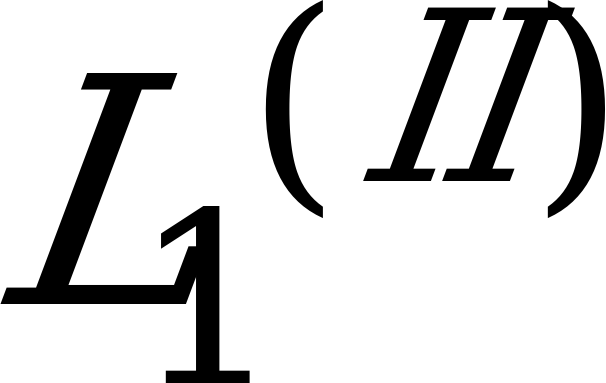Length of the first part of the LP analysis window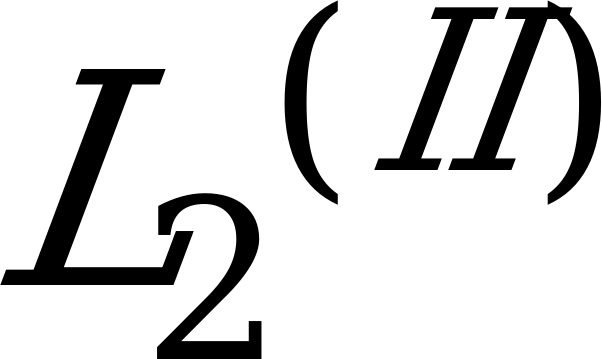Length of the second part of the LP analysis window

The auto‑correlations of the windowed speechLag window for the auto‑correlations (60 Hz bandwidth expansion)The bandwidth expansion in Hz

The sampling frequency in Hz

The modified (bandwidth expanded) auto‑correlations

The prediction error in the ith iteration of the Levinson algorithm

The ith reflection coefficient

The jth direct form coefficient in the ith iteration of the Levinson algorithm

Symmetric LSF polynomial

Antisymmetric LSF polynomial

Polynomial with root eliminated

Polynomial with root eliminatedThe line spectral pairs (LSPs) in the cosine domain

An LSP vector in the cosine domain

The quantified LSP vector at the ith subframe of the frame nThe line spectral frequencies (LSFs)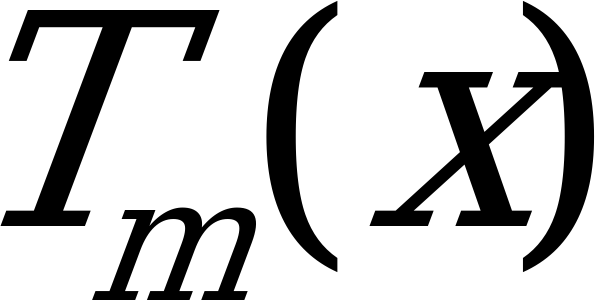Ath order Chebyshev polynomial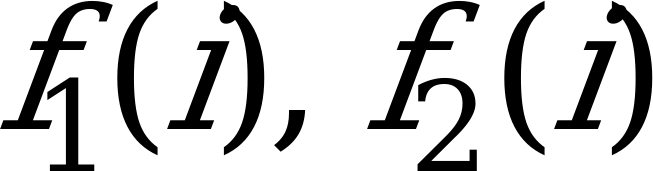The coefficients of the polynomialsand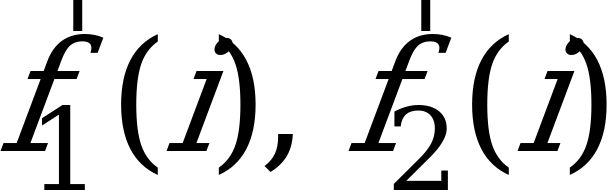The coefficients of the polynomials and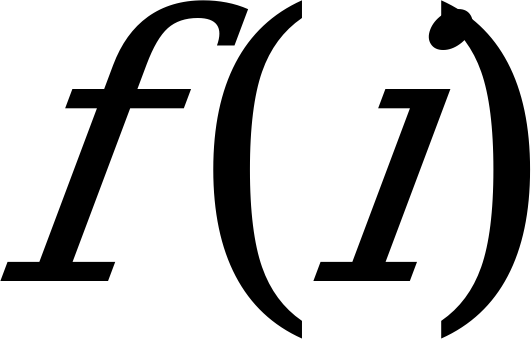The coefficients of either or

Sum polynomial of the Chebyshev polynomials

Cosine of angular frequency

Recursion coefficients for the Chebyshev polynomial evaluation

The line spectral frequencies (LSFs) in Hz

The vector representation of the LSFs in Hz

, The mean‑removed LSF vectors at frame n

, The LSF prediction residual vectors at frame n

The predicted LSF vector at frame n

The quantified second residual vector at the past frame

The quantified LSF vector at quantization index k

The LSP quantization errorLSP‑quantization weighting factors

The distance between the line spectral frequencies and

The impulse response of the weighted synthesis filter

The correlation maximum of open‑loop pitch analysis at delay k

The correlation maxima at delays

The normalized correlation maxima and the corresponding delays

The weighted synthesis filter

The numerator of the perceptual weighting filter

The denominator of the perceptual weighting filter

The integer nearest to the fractional pitch lag of the previous (1st or 3rd) subframeThe windowed speech signal

The weighted speech signal

Reconstructed speech signal

The gain‑scaled post‑filtered signal

Post‑filtered speech signal (before scaling)

The target signal for adaptive codebook search

, The target signal for algebraic codebook search

The LP residual signal

The fixed codebook vector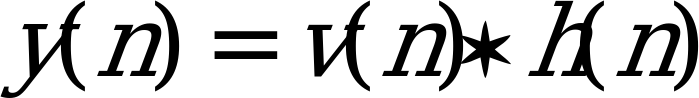The filtered adaptive codebook vector

The past filtered excitation

The excitation signal

The gain‑scaled emphasized excitation signalThe best open‑loop lagMinimum lag search value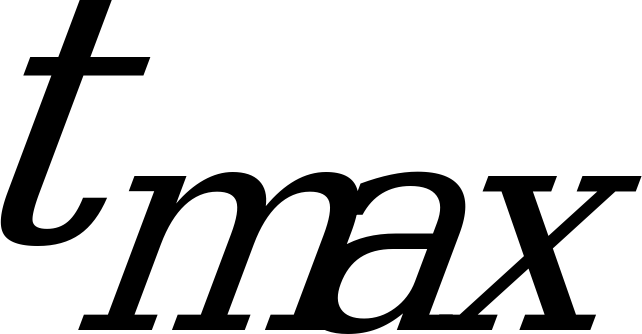Maximum lag search value

Correlation term to be maximized in the adaptive codebook search

The FIR filter for interpolating the normalized correlation term

The interpolated value of for the integer delay k and fraction t

The FIR filter for interpolating the past excitation signal to yield the adaptive codebook vector

Correlation term to be maximized in the algebraic codebook search at index k

The correlation in the numerator of at index k

The energy in the denominator of at index k

The correlation between the target signal and the impulse response , i.e., backward filtered target

The lower triangular Toepliz convolution matrix with diagonal and lower diagonals

The matrix of correlations of

The elements of the vector d

The elements of the symmetric matrix

The innovation vector

The correlation in the numerator ofThe position of the ith pulse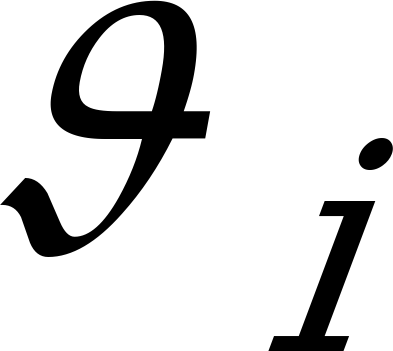The amplitude of the ith pulse

The number of pulses in the fixed codebook excitation

The energy in the denominator of

The normalized long‑term prediction residual

The signal used for presetting the signs in algebraic codebook search

The sign signal for the algebraic codebook search

Sign extended backward filtered target

, The fixed codebook vector convolved with

The mean‑removed innovation energy (in dB)The mean of the innovation energy

The predicted energy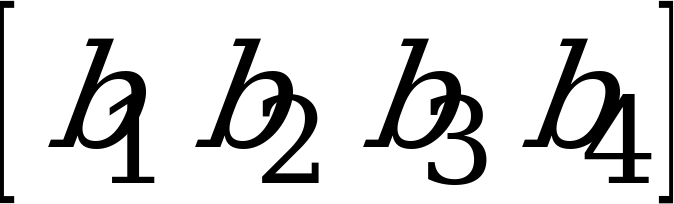The MA prediction coefficients

The quantified prediction error at subframe k

The mean innovation energy

The prediction error of the fixed‑codebook gain quantization

The quantization error of the fixed‑codebook gain quantization

The states of the synthesis filter

The perceptually weighted error of the analysis‑by‑synthesis search

The gain scaling factor for the emphasized excitation

The fixed‑codebook gain

The predicted fixed‑codebook gain

The quantified fixed codebook gain

A correction factor between the gain and the estimated one

The optimum value for

Gain scaling factor

## 3.3 Abbreviations

For the purposes of the present document, the following abbreviations apply. Further GSM related abbreviations may be found in GSM 01.04 .

ACELP Algebraic Code Excited Linear Prediction

CELP Code Excited Linear Prediction

EFR Enhanced Full Rate

FIR Finite Impulse Response

FR Full Rate

HR Half Rate

ISPP Interleaved Single‑Pulse Permutation

LP Linear Prediction

LPC Linear Predictive Coding

LSF Line Spectral Frequency

LSP Line Spectral Pair

LTP Long Term Predictor (or Long Term Prediction)

MA Moving Average